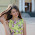## Pages

### Python-Basic Operators

Python language supports following type of operations:
[Arithematic, Comparison, Logical, Assignment, Conditional]

Arithematic operators are:

+ ,  - ,  * ,  / ,  % , ** (exponent) , // (division but digits after decimal in quotient is removed)

Comparison Operators:
• ' == ' Checks if the value of two operands are equal or not, if equal then condition becomes true.
• ' != ' Checks if the value of two operands are equal or not, if not equal then condition becomes true.
• ' <> ' is similar to ' != '
• ' > '  checks if the value of left operand is greater than right operand and, if yes then condition become true. Similarly there are comparison operators '<' , '>=', '<=' as in C.

Assignment Operators:

These operators assigns values to variables. They are :
=, +=, -=, *=, /=, %=, **=, //=
(functions similar to that in C)

Bitwise Operators:

Bitwise operator works on bits and perform bit by bit operation.
&      -bitwise AND
|        -bitwise OR
^      -bitwise XOR
~      - Binary Ones Complement Operator is unary and has the efect of 'flipping' bits.
<<   -binary leftshift operator
>>   -binary right shift operator

Logical Operators:

and     - Called Logical AND operator. If both the operands are true then then condition becomes true. For example:
if (a==4) and (b==6):
print (a+b)
Similarly there are or and not operators.

Membership Operators:

Python has membership operators, which test for membership in a sequence, such as strings, lists, or tuples. There are two membership operators.
They are:
in  - Evaluates to true if it finds a variable in the specified sequence and false otherwise.
for example:
a = 'vinod'

if ('n' in a):
print a

Since in string a there is a character 'n' it prints the string a.

The other membership operator is not in.
(just opposite of in)

Identity Operators:
(is , is not)
is - Evaluates to true if the variables on either side of the operator point to the same object and false otherwise.
For example:
a ="helloooo"
b ="helloooo"
if a is b:
print "strings are same!"
else:
print "strings are not same"
Since the both strings are same, it prints strings are same!

The other one is is not and it is just opposite of is.

Operator Precedence:

Highest precedence to lowest precedence:(from top to bottom)
**
~ + -
* / % //
+ -
<< >>
^ |
<= <> >=
<> == ~=
= %= /= //= -= += *= **=

#### 1 comment :

1.